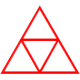# Circle and Square

Here’s another great geometry + algebra problem, posed by Megan Schmidt and pitched by Justin Aion to some students in his Geometry class.

Following is the problem as Justin posed it yesterday.Justin described the efforts of three of his students’ on his his ‘blog.  Following is my more generalized approach.  Don’t read further if you want to solve this problem for yourself!

My first instinct in any case like this is build it in a dynamic geometry package and play.  Using my TI-Nspire, without loss of generality, I graphed a circle centered at the origin, constructed a tangent segment at the bottom of the circle centered on the y-axis, and then used that segment to construct a square.  I recognized that the locus of the upper right corners of all such squares would form a line.That made it clear to me that for any circle, there was a unique square that intersected the circle three times as Megan had posed.

Seeing this and revealing its algebraic bias, my mind conceived an algebraic solution.  Assuming the radius of the circle is R, the equation of my circle is$x^2+y^2=R^2$ making the lower y-intercept of the circle$(0,-R)$.  That made$y=2x-R$ the locus line containing the upper right corner of the square.To find generic coordinates of the upper right corner of the square in terms of R, I just needed to solve the system of equations containing the circle and the line.  That’s easy enough to compute by hand if you can handle quadratic algebra.  That manipulation is not relevant right now, so my Nspire CAS’s version is:The output confirms the two intersections are$(0,-R)$ and the unknown at$\displaystyle \left( \frac{4R}{5} , \frac{3R}{5} \right)$.

Because of the horizontal symmetry of the square with respect to the y-axis, the system solution shows that the generic length of the side of the square is$\displaystyle 2\left( \frac{4R}{5} \right) = \frac{8R}{5}$.  The circle’s y-intercept at$(0,-R)$ means the generic diameter of the circle is$2R$.

Therefore, the generic ratio of the circle’s diameter to the square’s side length is$\displaystyle \frac{diameter}{side} = \frac{2R}{(8R)/5} = \frac{5}{4}$.

And this is independent of the circle’s radius!  The diameter of the circle is always$\frac{5}{4}$ of the square’s side.

CONCLUSION:

For Megan’s particular case with a side length of 20, that gives a circle diameter of 25, confirming Justin’s students’ solution.

Does anyone have a different approach?  I’m happy to compile and share all I get.

AN ASIDE:

While not necessary for the generalized solution, it was fun to see a 3-4-5 right triangle randomly appear in Quadrant 1.### 6 responses to “Circle and Square”

1.Tim

I like your algebraic approach. Two other ways? 1) Label the center of the circle. Make a vertical segment from there to the top of the square, and another from the circle’s center to the top right corner of the square. Now apply the Pythagorean Theorem. 2) Extend the two segments into diameters and use the “intersecting chords theorem.”

•chrisharrow

Nice, Tim. I should have seen your first method myself. The second is obvious now, but my brain always seems to “see” algebra first. I appreciate the alternatives!

2.Five Triangles

Our solution and commentary can be found here:
http://bit.ly/15TKNYC

3.travis

I’m off to Masters swim, but a cursory glance… 10*10 = x * 20
x=5, then 20+5=25….all this using perp. chords. Maybe you mention this. I will read the ‘solution’ later.

•chrisharrow

Much cleaner. Thanks. It also connects very nicely to a proof of the Pythagorean Theorem. See my next post.

4.chrisharrow

Mike Lawler (@mikeandallie) replied on Twitter with two more nice solutions he posted on YouTube at